## Excel UNIQUE Function

The guide demonstrates how to use the UNIQUE function and dynamic arrays in Excel to create unique values. You’ll learn how to use a basic formula to locate unique values inside a column or row, across many columns, and depending on criteria, among other things.

Excel 365’s introduction of the Excel UNIQUE formula altered everything! What was once considered rocket science becomes as simple as ABC. Now, you don’t need to be an expert in formulas to extract unique values from a range based on one or more criteria and organize the results alphabetically. All of this is accomplished via the use of straightforward formulae that anybody can understand and adapt to their own requirements.

## Notes on usage

The Excel UNIQUE function returns a list of values that are unique inside a range or array. As a consequence, a dynamic array of unique values is created. If this array is the end output (i.e. it is not passed to another function), array contents “spill” onto the worksheet into a range that updates automatically when new unique values are added or deleted from the source range.

The UNIQUE function accepts three arguments: an array, a by col parameter, and an exactly once argument. The first parameter, array, specifies the array or range from which unique values should be extracted. This is the only argument that is necessary. The second input, by col, specifies whether unique data are extracted by rows or columns. UNIQUE extracts unique values from rows by default. Set by col to TRUE or 1 to compel UNIQUE to extract unique data by column. The last option, exactly once, specifies the behavior for values that occur many times. By default, UNIQUE extracts all unique values, regardless of their number of appearances in the array. Set exactly once to TRUE or 1 to retrieve unique values that exist just once in the array.

## UNIQUE function in Excel

In Excel, the UNIQUE function produces a list of distinct values from a range or array. It works with any form of data, including text, numbers, dates, and timings.

The function falls within the category of Dynamic Arrays functions. As a consequence, a dynamic array is created that naturally overflows onto adjacent cells, either vertically or horizontally.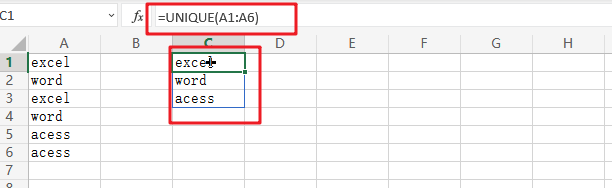The Excel UNIQUE function has the following syntax:

`=UNIQUE(array, [by col], [once exactly])`

Where:

• `Array` (needed) – the range or array from which unique values are to be returned.
• `By col`` (`optional) – a logical value specifying how data should be compared:
• `TRUE` – does cross-column comparisons.
• `FALSE` or omitted (default) – does cross-row comparisons.
• `Exactly once` (optional) – a logical value defining which values are regarded as unique:
• `TRUE` – yields values that only occur once, which corresponds to the database concept of uniqueness.
• `FALSE or omitted` (default) – returns the range or array’s distinct (different) values.

### Important note:

At the moment, the UNIQUE feature is only accessible in Microsoft Excel 365 and Excel 2021. Excel 2019, 2016, and older versions do not allow dynamic array formulae, and so do not have the UNIQUE function.

### Related Functions

• Excel Filter function
The FILTER function extracts matched records from a collection of data using one or more logical checks. The include argument specifies logical tests, which might encompass a wide variety of formula conditions.==FILTER(array,include,[if empty])…

## Extract Common Values in Two Lists

Just assume that you have two lists containing values/words in the few cells, and you want to extract the same or common values/words from the two lists into another separate list; then you might think that it’s not a big deal; because you would prefer to manually extract the same or common values/words from the two lists into another separate list without any need of the formula.

Then congratulations because you are thinking right, but let me include that it would be a big deal to extract the same or common values/words from the two lists including multiple cells into another separate list and doing it manually would be a foolish attempt, because there are 90% chances that you would 100% get tired of it and would never complete your work on time.

But don’t be worry about it because after carefully reading this article, extracting the same or common values/words from the two lists into another separate list would become a piece of cake for you.

So let’s dive into the article to take you out of this fix.

## General Formula

The Following formula would help you compare and extract the same or common values/words from the two lists into another separate list.

`=FILTER(Table1,COUNTIF(Table2, Table1))`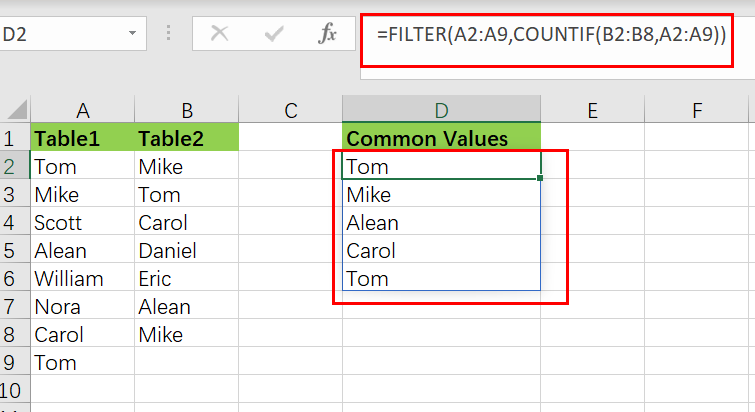This Formula is based on the FILTER and COUNTIF function, where the` Table1 (A2:A7)` and `Table2 (B2:B7)` are the named ranges, the table in the range` D2:D7` is the common list containing the common elements or values by comparing both Table1 and Table2.

## Syntax Explanations:

Before going into the explanation of the formula for getting the work done efficiently, we must understand each syntax which would make it easy for you that how each syntax contributes to executing the same or common values/words from the two lists into another separate list:

• `Filter`: This function contributes to narrowing down or filtering out a range of data on the user-defined criteria.
• `COUNTIF`: It is a statistical function that contributes to counting the number of cells to meet specific criteria
• `List`: In this formula, the list represents the two lists present in the excel worksheet to execute the common values
• `Comma symbol` (,): In Excel, this comma symbol acts as a separator that helps to separate a list of values.
• `Parenthesis` (): The core purpose of this Parenthesis symbol is to group the elements and to separate them from the rest of the elements.

## Let’s See How This Formula Works:

The FILTER function takes an array of values as input and an “`include`” parameter to filter the array based on a logical expression or value.

The array is provided in this example as the named range “Table1“, which contains all values in the range A2:A7. The COUNTIF function, which is nested inside FILTER, provides the included argument:

`=FILTER(Table1,COUNTIF(Table2, Table1))`COUNTIF is set up with Table1 as criteria and Table2 as the range. Because if the eight criteria values are given to the  COUNTIF, then as an array, it would also return eleven results like the following:

`{1;1;0;1;0;1;0;1;0;1;1}`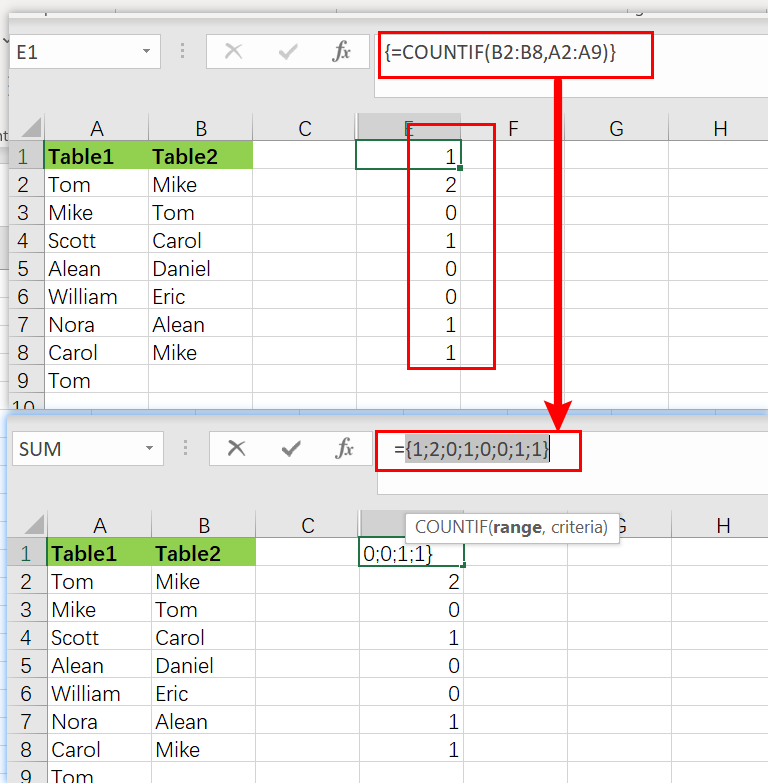Keep it into your notice that the 1’s correspond to the Table2 items, which also appear in the Table1.

As with the aid of “include” argument this array is delivered to the FILTER function directly:

`=FILTER(Table1,{1;1;0;1;0;1;0;1;0;1;1})`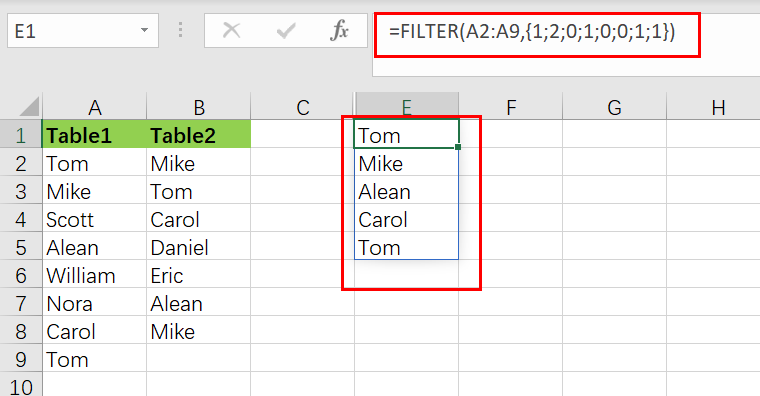Using the values provided by COUNTIF, the FILTER function efficiently filters the Table1. The values except zero are preserved, and the values associated with zero are removed.

The list spread into the range D2:D7 is the final result consisting of an array of values common in both Table1and Table2.

## More Examples

The raw results from COUNTIF are used as the filter in the above algorithm. This works because Excel considers any non-zero number to be TRUE and any zero value to be FALSE. If COUNTIF returns a count larger than one, the filter will continue to function normally.

You can use “>0” to force TRUE and FALSE results explicitly, like as follows:

`=FILTER(Table1,COUNTIF(Table2,Table1)>0)`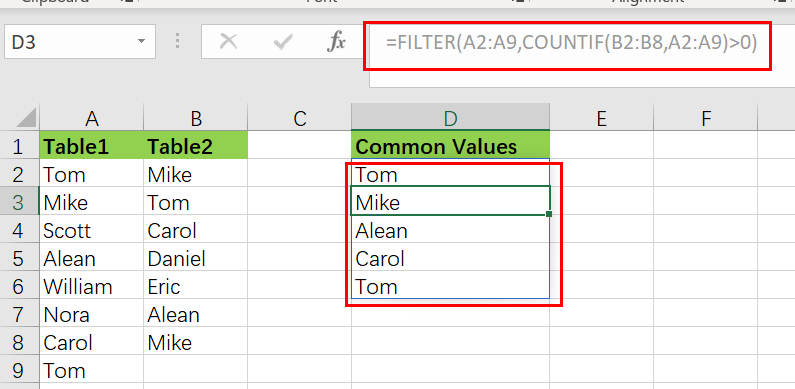### Remove duplicates From Common Values

Nest the formula inside the UNIQUE function to remove the duplicates, just like as follows:

`=UNIQUE(FILTER(Table1,COUNTIF(Table2,Table1)))`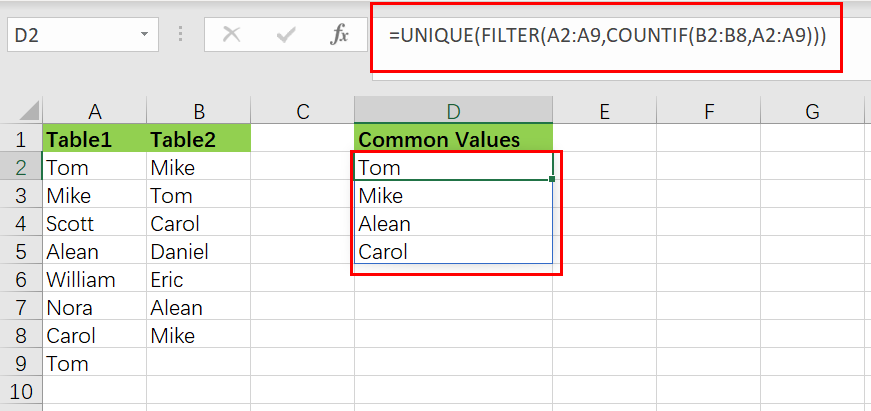### Sort Common Values

Just nest the formula in the SORT function to sort results:

`=SORT(UNIQUE(FILTER(Table1,COUNTIF(Table2,Table1))))`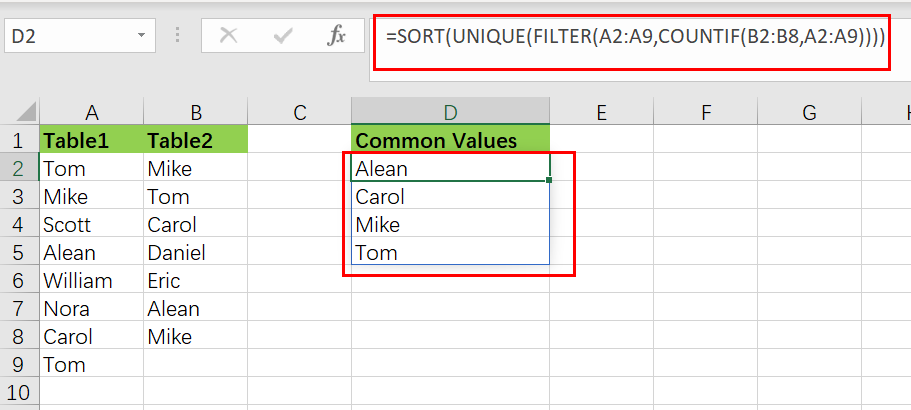### Extract values missing from Table2

You can reverse the logic for getting the output values in Table1 missing from Table2, like in the follows:

```=FILTER(Table1,COUNTIF(Table2,Table1)=0)
```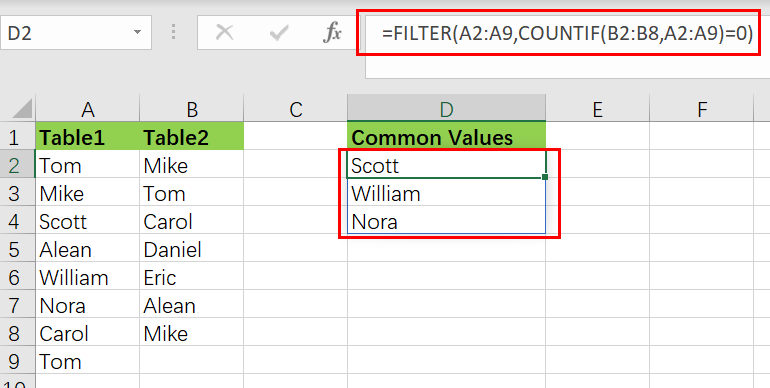### Related Functions

• Excel COUNTIF function
The Excel COUNTIF function will count the number of cells in a range that meet a given criteria. This function can be used to count the different kinds of cells with number, date, text values, blank, non-blanks, or containing specific characters.etc.= COUNTIF (range, criteria)…
• Excel Filter function
The FILTER function extracts matched records from a collection of data using one or more logical checks. The include argument specifies logical tests, which might encompass a wide variety of formula conditions.==FILTER(array,include,[if empty])…
• Excel Sort function
The SORT function in Excel sorts the contents of an array or range alphabetically or numerically by columns or rows.The syntax:=SORT(array, [sort index],) …
• Excel UNIQUE function
The guide demonstrates how to use the UNIQUE function and dynamic arrays in Excel to create unique values.The syntax:=UNIQUE(array, [by col], [once exactly]) …

## How to Count Unique Dates in Excel

This post will guide you how to count unique dates in a given range in Excel 2013/2016 or Excel office 365. How do I count the unique dates in a list of dates with some duplicate dates using a formula in Excel. And you can do this by a formula based on the SUMPRODUCT function and the COUNTIF function in an older version of Excel 2013/2016. You can also do it using UNIQUE function in the latest Excel 365.

Assuming you have a list of data range B1:B6 in your worksheet and you wish to count the number of unique dates using a formula. And the below introduction will show you the ways to achieve the result.

## Count Unique Dates in Old Version of Excel

If you are using older version of Excel, for example, Excel 2013 or Excel 2016, and you can use the COUNTIF function in combination with the SUMPRODUCT function to count unique dates with a formula like this:

`=SUMPRODUCT(1/COUNTIF(B1:B6,B1:B6))`

Let’s See How This Formula Works:

`=COUNTIF(B1:B6,B1:B6)`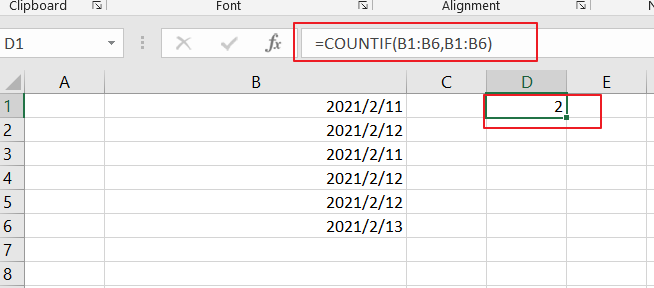The COUNTIF function will compare each date in the same data range and returns an array results that contain a count for every data in the given range. The result array is like this:

`{2;3;2;3;3;1}`
`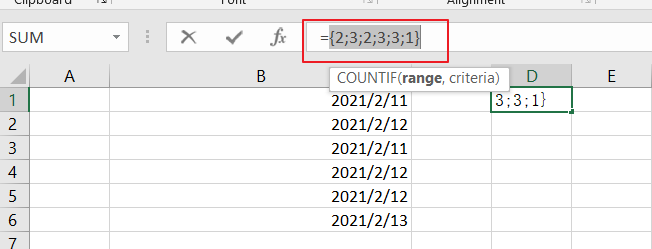`

Then you need to divide this array result by number 1 to return an array of fractional values like this:

`=1/COUNTIF(B1:B6,B1:B6)`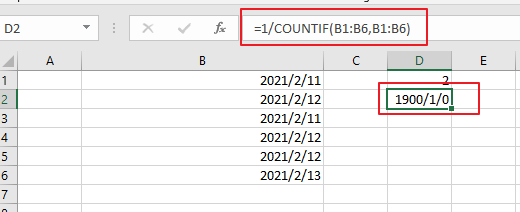`={0.5;0.333333333333333;0.5;0.333333333333333;0.333333333333333;1}`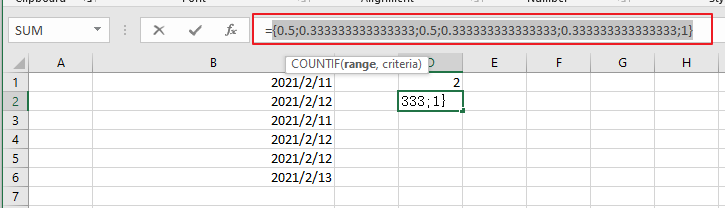Finally, you still need to add up the items in the above array. And you can use the SUMPRODUCT function to sum up the items in that array list. And it will return the total unique dates as 3.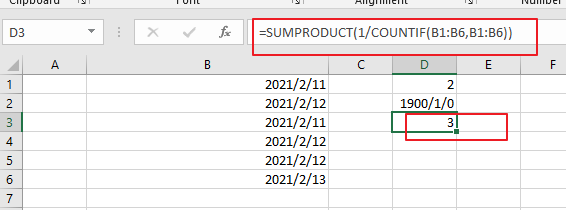You can also use another array formula based on the SUM Function and the FREQUENCY function to achieve the result of counting unique dates in the given date range. Like below:

`=SUM(--(FREQUENCY(B1:B6,B1:B6)>0))`

Then you need to put this formula into a blank cell and press “Ctrl +Shift +Enter” to make it as array formula.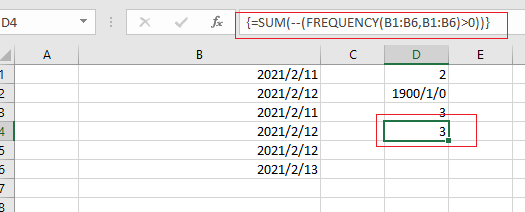Count Unique Dates in Excel 365

If you are working in Excel 365 and you can use the UNIQUE function in combination with the COUNT function to count unique dates in the given range cells in Excel.

The generic formula is like this:

`=COUNT(UNIQUE(B1:B6)`

The UNIQUE function can be used to extract a list of unique dates from a given range B1:B6, and then using the COUNT function to count numeric values. And if you wish to count both numeric and text values, you can replace the COUNT function with COUNTA function.

### Related Functions

• Excel SUMPRODUCT function
The Excel SUMPRODUCT function multiplies corresponding components in the given one or more arrays or ranges, and returns the sum of those products.The syntax of the SUMPRODUCT function is as below:= SUMPRODUCT (array1,[array2],…)…
• Excel COUNTIF function
The Excel COUNTIF function will count the number of cells in a range that meet a given criteria. This function can be used to count the different kinds of cells with number, date, text values, blank, non-blanks, or containing specific characters.etc.= COUNTIF (range, criteria)…
• Excel SUM function
The Excel SUM function will adds all numbers in a range of cells and returns the sum of these values. You can add individual values, cell references or ranges in excel.The syntax of the SUM function is as below:= SUM(number1,[number2],…)…
• Excel COUNT function
The Excel COUNT function counts the number of cells that contain numbers, and counts numbers within the list of arguments. It returns a numeric value that indicate the number of cells that contain numbers in a range…
• Excel FREQUENCY function
TThe Excel FREQUENCY function calculates how often values occur within a range of values. And it will return a vertical array of numbers.The syntax of the FREQUENCY function is as below:= FREQUENCY (data_array, bins_array)…
• Excel COUNTA function
The Excel COUNTA function counts the number of cells that are not empty in a range. The syntax of the COUNTA function is as below:= COUNTA(value1, [value2],…)…
• Excel UNIQUE function
The guide demonstrates how to use the UNIQUE function and dynamic arrays in Excel to create unique values.The syntax:=UNIQUE(array, [by col], [once exactly]) …